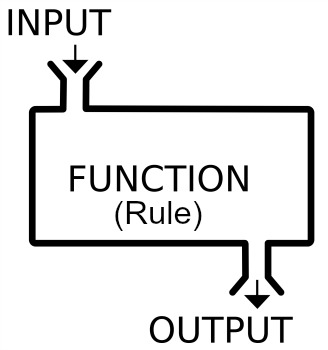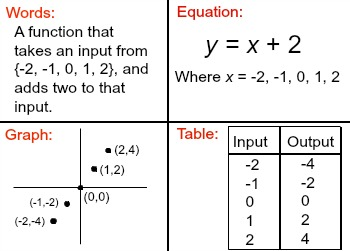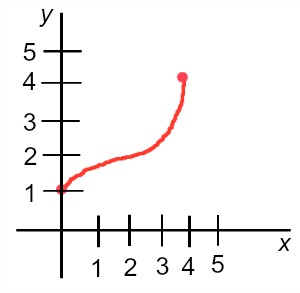# What is the Range of a Function? - Definition & Examples

Instructor: Mervin Clyde Cananes
The range of a function refers to the entire set of all possible output values of the dependent variable. Explore more on the concept of the range of a function and learn how to find it through analyzing some examples in this lesson. Updated: 04/12/2022

## Functions, Inputs, and Outputs

Before getting to the range of a function let's have a quick review of functions and their inputs and outputs. To say that 'a is a function of b', is the same as saying that 'a is determined by b'. For instance, consider a drink menu at a fast food restaurant. Assume a small drink is \$1.50, a medium drink is \$2.50, and a large drink is \$3.50. Notice that the price of the drink is determined by the size of the drink. Therefore, the price of a drink is a function of the size.

A function can be thought of as a rule that assigns a set of inputs to a set of outputs. In our example, the inputs would be the drink size, and the outputs would be the price. Therefore, our inputs are {small, medium, large}, and our outputs are {\$1.50, \$2.50, \$3.50}.In a function, it is imperative that each input is assigned to only one output. The reason for this is because if an input were assigned to two different outputs, then given the input, we could not determine the output. Given an input, we must be able to determine the output. Thus, each input of a function has only one output.

A function can be represented in different ways. We can use words to represent a function, as we did in our drink menu example. We can also use an equation, a graph, or a table to represent a function.## Definition of the Range of a Function

As we said, functions have inputs and outputs. The inputs are what we put in the function, and the outputs are what come out. We call the set of outputs of a function the range of a function. For example, consider the function defined by the rule that we take an input and raise it to the third power. This can be represented in equation form as y = x^3, and when this function is given input values of {-2, -1, 0, 1, 2}, we can find the corresponding outputs by plugging those inputs in for 'x' in the equation.

For instance, if we input -2, we have y = (-2)^3 = -8, so when the input is -2, the output is -8, and -8 is in our range. When we find each of the corresponding outputs to our inputs, we have our range. In our example, the range is {-8, -1, 0, 1, 8}, because these are the outputs corresponding to -2, -1, 0, 1, and 2 respectively.

## Finding Range of a Function

To find the range of a function, we simply need to find the functions outputs, based on its inputs. As we mentioned, functions can be represented in different ways. Let's look at some different examples of finding the range of a function for different representations of a function.

1.First, let's consider our initial drink menu example. We described this function using words. The function's rule assigns a small drink to \$1.50, a medium drink to \$2.50, and a large drink to \$3.50. The inputs are the drink size, and the outputs are the drink price. The range of this function is the set of all the outputs. Therefore, the range for this function is the set of all outputs, or {\$1.50, \$2.50, \$3.50}.

2.Consider the following function represented in the table.

Input Output
-3 -6
-2 -4
-1 -2
0 0
1 2
2 4
3 6

By definition the range is the set of all the outputs of a function, so to find the range, we simply list the outputs {-6, -4, -2, 0, 2, 4, 6}.

3.Observe this graph:To unlock this lesson you must be a Study.com Member.

### Register to view this lesson

Are you a student or a teacher?

Back

### Resources created by teachers for teachers

Over 30,000 video lessons & teaching resources‐all in one place.Video lessonsQuizzes & WorksheetsClassroom IntegrationLesson Plans

I would definitely recommend Study.com to my colleagues. It’s like a teacher waved a magic wand and did the work for me. I feel like it’s a lifeline.

Jennifer B.
TeacherCreate an account to start this course today
Used by over 30 million students worldwide